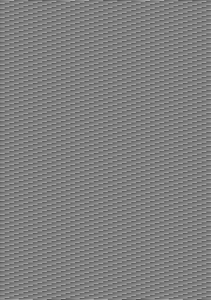Convert a NumPy array to an image

• Last Updated : 02 Sep, 2020

NumPy Or numeric python is a popular library for array manipulation. Since images are just an array of pixels carrying various color codes. NumPy can be used to convert an array into image. Apart from NumPy we will be using PIL or Python Image Library also known as Pillow to manipulate and save arrays.

Approach:

1. Create a numpy array.
2. Reshape the above array to suitable dimensions.
3. Create an image object from the above array using PIL library.
4. Save the image object in a suitable file format.

Below is the implementation:

Python3

 # Python program to convert# numpy array to image  # import required librariesimport numpy as npfrom PIL import Image as im  # define a main functiondef main():      # create a numpy array from scratch    # using arange function.    # 1024x720 = 737280 is the amount     # of pixels.    # np.uint8 is a data type containing    # numbers ranging from 0 to 255     # and no non-negative integers    array = np.arange(0, 737280, 1, np.uint8)          # check type of array    print(type(array))          # our array will be of width     # 737280 pixels That means it     # will be a long dark line    print(array.shape)          # Reshape the array into a     # familiar resoluition    array = np.reshape(array, (1024, 720))          # show the shape of the array    print(array.shape)      # show the array    print(array)          # creating image object of    # above array    data = im.fromarray(array)          # saving the final output     # as a PNG file    data.save('gfg_dummy_pic.png')  # driver codeif __name__ == "__main__":      # function call  main()

Output:

<class 'numpy.ndarray'>
(737280,)
(1024, 720)
[[  0   1   2 ... 205 206 207]
[208 209 210 ... 157 158 159]
[160 161 162 ... 109 110 111]
...
[144 145 146 ...  93  94  95]
[ 96  97  98 ...  45  46  47]
[ 48  49  50 ... 253 254 255]]gfg_dummy_pic.png 1024 x 720

Note: Every array can’t be converted into an image because each pixel of an image consist of specific color code and if the given array is not in a suitable format the libraries won’t be able to process it Properly.

My Personal Notes arrow_drop_up